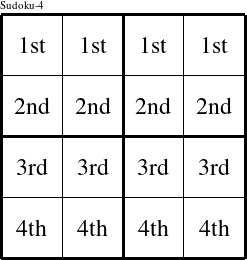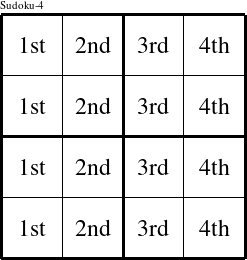#### Printable version

Detailed instructions for working a group puzzle of type 'Kurt'

This is a signature puzzle named 'Kurt'

The 4 elements in each group of this type of puzzle are: K, U, R, and T.

Every group in the puzzle must contain exactly this set of elements.

####In a Kurt puzzle, each row is a group numbered as shown in the figure above.

####In a Kurt puzzle, each column is a group numbered as shown in the figure above.

####In a Kurt puzzle, each 2x2 square is a group numbered as shown in the figure above.